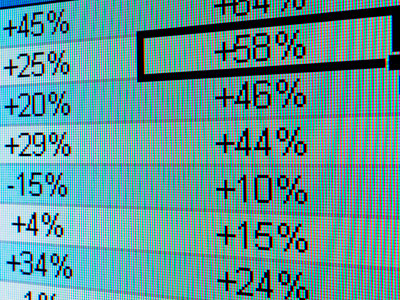# Models 1

This Computer Science quiz is called 'Models 1' and it has been written by teachers to help you if you are studying the subject at middle school. Playing educational quizzes is a fabulous way to learn if you are in the 6th, 7th or 8th grade - aged 11 to 14.

It costs only \$12.50 per month to play this quiz and over 3,500 others that help you with your school work. You can subscribe on the page at Join Us

Spreadsheets are a type of model in computer science. In the field of computer science, modeling is an important topic. And we're not talking about catwalk modeling!

See how much knowledge you have by playing our first Models quiz which is about using spreadsheets and rules to create models or simulations.

1.
Spreadsheets are applications designed to .......
do the same job as databases only they are cheaper
give you a large desktop to work on
help accountants
perform calculations on data in a convenient way
2.
Typical uses of spreadsheets would not include .......
creating invoices
doing basic calculations on a few numbers
producing charts and graphs
simple databases
A simple calculator would be adequate
3.
The work space is divided into rectangles called .......
bells
cells
sells
shells
4.
Each cell may contain .......
numbers and text and formulae at the same time
numbers and text at the same time
numbers only
numbers or text or formulae
Each cell should only contain one type of data at a time
5.
Cells are organized in rows and .......
boxes
columns
layers
shelves
6.
A cell is identified by the row and column it is in. They are .......
its identifier
its zip code
the cell location
Cell B2 is found in column B row 2
7.
What do we call a cell being worked on?
Active cell
Calc cell
Current cell
Live cell
8.
A cell can contain a 'formula'. A formula is .......
an instruction telling the spreadsheet what actions to take
an instruction telling the user what actions to take
a mathematical equation
None of these
9.
A formula in a cell usually begins with a .......
#
~
^
=
Note - not all spreadsheets use = this way
10.
A cell can be referred to in a formula using a relative or .......